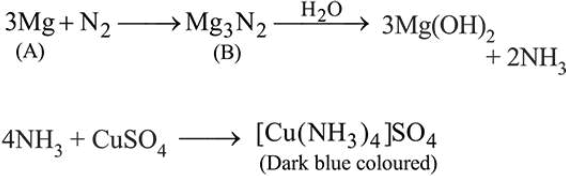# A metal (A) on heating in nitrogen gas gives compound B.

Question:

A metal (A) on heating in nitrogen gas gives compound B.

B on treatment with $\mathrm{H}_{2} \mathrm{O}$ gives a colourless gas which when passed through $\mathrm{CuSO}_{4}$ solution gives a dark blueviolet coloured solution. A and B respectively, are:

1. $\mathrm{Na}$ and $\mathrm{NaNO}_{3}$

2. $\mathrm{Na}$ and $\mathrm{Na}_{3} \mathrm{~N}$

3. $\mathrm{Mg}$ and $\mathrm{Mg}_{3} \mathrm{~N}_{2}$

4. $\mathrm{Mg}$ and $\mathrm{Mg}\left(\mathrm{NO}_{3}\right)_{2}$

Correct Option: , 3

Solution: